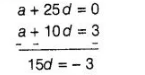# The 26 th, 11 th and the last terms of an AP are,

Question:

The 26 th, 11 th and the last terms of an AP are, 0,3 and $\frac{-1}{6}$, respectively. Find the common difference and the number of terms.

Solution:

Let the first term, common difference and number of terms of an AP are a, d and n, respectively.

We know that, if last term of an AP is known, then

$l=a+(n-1) d$ ...(i)

and $n$th term of an AP is

$T_{n}=a+(n-1) d$ ...(ii)

Given that, 26 th term of an $\mathrm{AP}=0$

$\Rightarrow \quad T_{26}=a+(26-1) d=0 \quad$ [from Eq. (i)]

$\Rightarrow \quad a+25 d=0$ ....(iii)

11 th term of an $A P=3$

$\Rightarrow \quad T_{11}=a+(11-1) d=3$ [from Eq. (ii)]

$\Rightarrow \quad a+10 d=3$ $\ldots$ (iv)

and last term of an $A P=-1 / 5$

$\Rightarrow \quad l=a+(n-1) d \quad$ [from Eq. (1)]

$\Rightarrow \quad-1 / 5=a+(n-1) d \quad \ldots(v)$

Now, subtracting Eq. (iv) from Eq. (iii),$\Rightarrow \quad d=-\frac{1}{5}$

Put the value of $d$ in Eq. (iii), we get

$a+25\left(-\frac{1}{5}\right)=0$

$\Rightarrow \quad a-5=0 \Rightarrow a=5$

Now, put the value of $a_{1} d$ in Eq. (v), we get

$-1 / 5=5+(n-1)(-1 / 5)$

$\Rightarrow \quad-1=25-(n-1)$

$\Rightarrow \quad-1=25-n+1$

$\Rightarrow \quad n=25+2=27$

Hence, the common difference and number of terms are $-1 / 5$ and 27 , respectively.